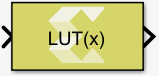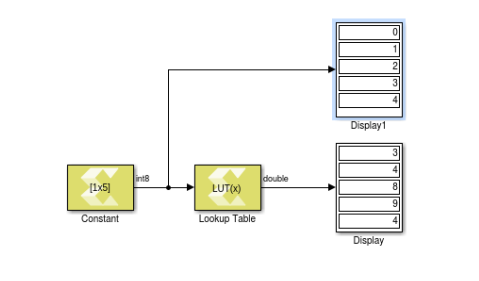# Lookup Table - 2022.1 English

## Vitis Model Composer User Guide (UG1483)

Document ID
UG1483
Release Date
2022-05-26
Version
2022.1 English

Perform one-dimensional lookup operation with an input index.

## Library

Lookup Tables## Description

The Lookup Table block implements a simple read-only memory block with an input index. The block maps input to an output value by looking up a table of values you define with a Table data parameter.

The input value is used as a zero-based index into the table data. The Input bias parameter is an offset to the index value (to support negative indexing). A When input is out of range parameter lets you specify the behavior of the block if the index value exceeds the valid table size range.

Note: If the table size is not an exact power of 2, the block incurs additional hardware cost when it is implemented in a Xilinx device, due to a remainder calculation on the index.
Figure 1. Lookup Table BlockIn the example above, the Table data setting for the Lookup Table block is [7 3 4 8 9 4 1 5] with an input bias of 1.

## Data Type Support

The Lookup Table block accepts the following data types to represent scalar index value: int8, uint8, int16, uint16, int32, uint32, and fixed-point type. Fixed-point inputs is shifted appropriately to generate an integer index.

The output data type is same as the Table data parameter type. Inputs for indexing must be real, but table data can be complex.

## Parameters

Table Data

This parameter accepts a 1-D vector of table values. The size of the vector determines the valid index range for the input index. The data will be explicitly converted into the type specified in the Output data type parameter. If the input index exceeds table size and Saturate at table ends is specified for the When input is out of range parameter, then index value is saturated to either top or bottom of table size range. If Wrap around is specified for the When input is out of range parameter, the index is wrapped into the valid table size range.

Note: Large tables should be defined via a Simulink workspace variable due to space limitations in the block dialog box.

Input bias

This parameter is an offset into the table data that will be added to the index input. This makes it possible to use negative indices and perform look up operation.

When input is out of range

This parameter will guard the index value if it exceeds the valid table size range.

Following are the settings for the When input is out of range parameter.

Table 1. When Input Is Out of Range Parameter
Setting Description
Saturate at table ends If index value exceeds the valid table size range, then index value is saturated to either top or bottom of table size range, depending on the overflow direction.
Wrap around If index value exceeds the valid table size range, then index value is wrapped into the valid table size range.

Output data type

Specifies the output data type.

Following are settings for the Output data type parameter.

Table 2. Output Data Type Parameter
Setting Description
double double precision floating-point
single single precision floating-point
int8 8-bit signed integer
uint8 8-bit unsigned integer
int16 16-bit signed integer
uint16 16-bit unsigned integer
int32 32-bit signed integer
uint32 32-bit unsigned integer
boolean boolean
fixed fixed-point
half half precision floating-point
data type expression

A string that specifies the output data type. See "Working with Data Type Expression" in the Vitis Model Composer User Guide (UG1483).